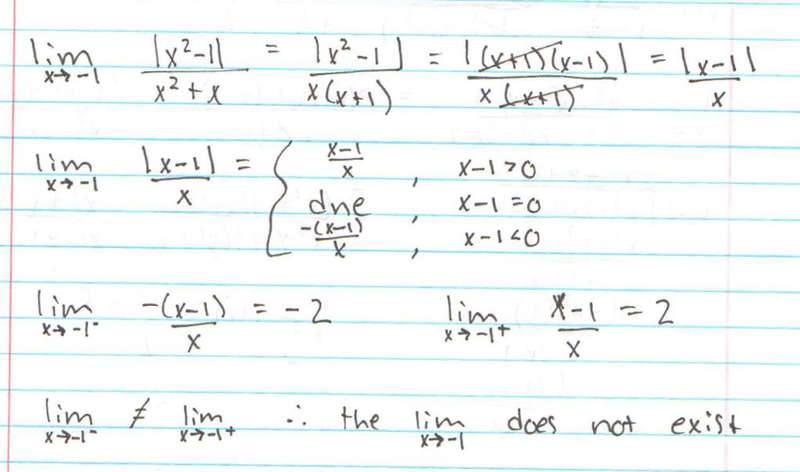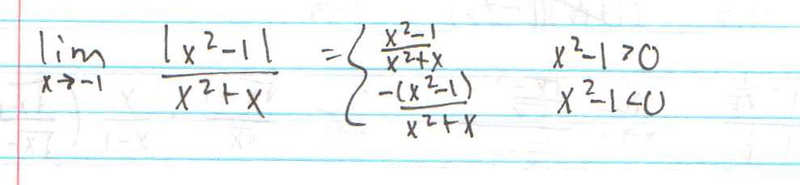# Basic limit involving absolute value

## Homework Statement

lim as x$\rightarrow$-1 = $\frac{|x^{2}-1|}{x^{2}+x}$

N/a

## The Attempt at a Solution

Tried to write this as a piecewise function, but I got lost.

Hi wvcaudill2!Calculate the right-sided and the left-sided limits separately. That way, you could get rid of those nasty absolute value signs!

Ok, from the left-hand side of the limit, I got -2, and from the right-hand side I got 2, and when x-1=0 I got "does not exist." Is this right?

Ok, from the left-hand side of the limit, I got -2, and from the right-hand side I got 2,

The correct solution should be those two switched. From the left side, you should get 2. From the right side, you should get -2. Check your signs.

and when x-1=0 I got "does not exist."

What in earth do you mean with this??

I do not see how they should be switched. Can you explain your process in more detail?

I do not see how they should be switched. Can you explain your process in more detail?

Well, show your work, and I'll show you what went wrong...You can't just eliminate the x+1 in your first line. Why not? Because it sits inside an absolute value sign. This will cause sign errors.

The absolute first thing you should do is eliminate the absolute value signs around $x^2-1$. If x comes from the left, then this expression is positive, so you can drop the absolute valuie signs. If x comes from the right, then it is negative, so you'll have to write a minus sign in front.

Im still not sure how it is you are coming to the statement that "if x comes from the left, then this expression is positive." Can you explain how you are getting this?

Here is what I have now:Im still not sure how it is you are coming to the statement that "if x comes from the left, then this expression is positive." Can you explain how you are getting this?

Here is what I have now:Well, if $x\leq -1$, then $x^2-1\geq 0$. This is easily seen by drawing the parabola for $x^2-1$.

Ok, I see what you are saying now, but when I graphed the parabola, I also saw that coming from the right-hand side, the values of x were also positive up until x=1.

Ok, I see what you are saying now, but when I graphed the parabola, I also saw that coming from the right-hand side, the values of x were also positive up until x=1.

The parabola is negative between -1 and 1: http://www.wolframalpha.com/input/?i=y%3Dx^2-1

Im still not seeing the big picture here. Can you write out exactly what this problem should look like with all the steps at least partially worked out?